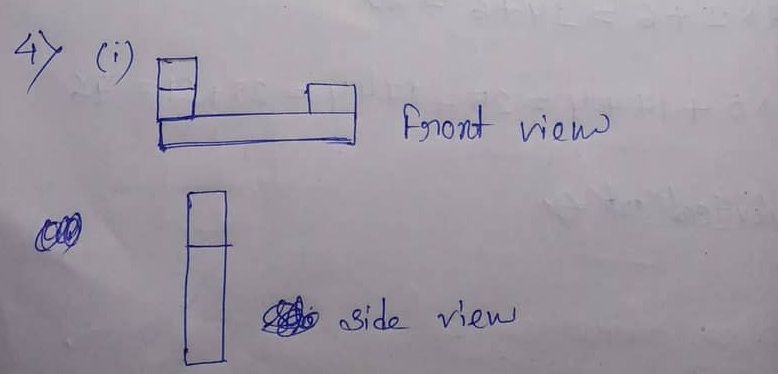# ML Aggarwal CBSE Solutions Class 7 Math Fifteenth Chapter Visualising Solid Shapes Exercise 15.3

### ML Aggarwal CBSE Solutions Class 7 Math 14th Chapter Visualising Solid Shapes Exercise 15.3

(1) (i) 5 x 3 x 2 = 15 x 2 = 30

(ii) 7 x 2 + 6 = 14 + 6 = 20

(iii) 5 x 5 + 17 + 4 = 25 + 17 + 4 = 25 + 21 = 46

(2)

 Vertical cut Horizontal cut (a) A brick Rectangle Rectangle (b) A round apple Circle Circle (c) A die Square Square (d) A circular pipe Rectangle Circle (e) An ice cream Triangle Circle (f) A square pyramid Triangle Square

(3) (a) (1) Side (2) Top (3) Front

(b) (1) Front (2) Side (3) Top (Front)

(c) (1) Top (2) Side (3) Front

(d) (1) front (Side) 92) Side (front (3) Top

(e) (1) Top (2) Side (3) FrontUpdated: November 22, 2019 — 1:26 pm